Graphs and Charts

Graphs and Charts

Besides grouping, another method for organizing and summarizing data is to draw a picture of some kind. The old saying "a picture is worth a thousand words" has particular relevance in statistics -- a graph or chart of a data set often provides the simplest and most efficient display.

Histograms, dotplots, and stem- and- leaf diagrams are designed for use with quantitative data.

Frequency Histograms

A graph that displays the classes on the horizontal axis and the frequencies of the classes on the vertical axis. The frequency of each class is represented by a vertical bar whose height is equal to the frequency of the class.

Relative-Frequency Histogram

A graph that displays the classes on the horizontal axis and the relative frequencies on the classes on the vertical axis. The relative frequency of each class is represented by a vertical bar whose height is equal to the relative frequency of the class.

Relative- frequency histograms are better than frequency histograms for comparing two data sets. The same vertical scale is used for all relative-frequency histograms -- a minimum of 0 and a maximum of 1 -- making direct comparison easy. In contrast, the vertical scale of a frequency histogram depends on the number of observations, making comparison more difficult.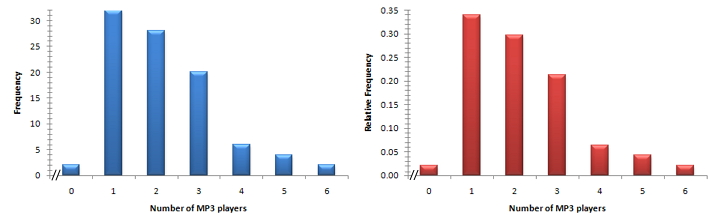Example of a frequency histogram and a relative-frequency histogram

Another type of graphical display for quantitative data is the dotplot. Dotplots are particularly useful for showing the relative positions of the data in a data set or for comparing two or more data sets.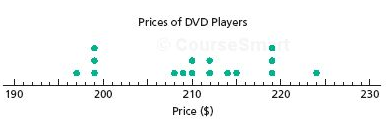Example of a dotplot diagram

Statisticians continue to invent ways to display data. One method, developed in the 1960s by the late Professor John Tukey of Princeton University, is called a stem- and- leaf diagram. This ingenious diagram is often easier to construct than either a frequency distribution or a histogram and generally displays more information.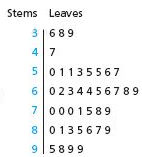Example of a stem-and- leaf diagram.

Steps to construct a Stem-and-Leaf Diagram

1. Think of each observation as a stem, consisting of all but the rightmost digit, and a leaf, the rightmost digit.
2. Write the stems from smallest to largest in a vertical column to the left of a vertical rule
3. Write each leaf to the right of the vertical rule in the row that contains the appropriate stem.
4. Arrange the leaves in each row in ascending order.

We use different techniques for qualitative data: two common methods are the pie chart and the bar graph.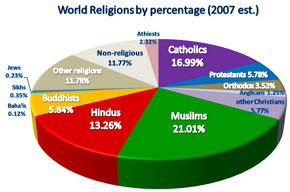Example of a pie graph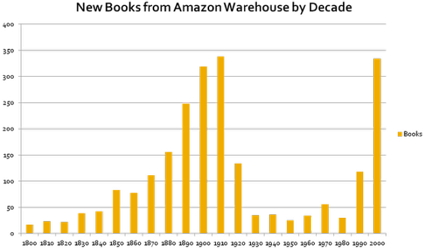Example of a bar graph

A bar graph is like a histogram. However, to avoid confusing bar graphs and histograms, the bars in a bar graph are positioned so that they do not touch each other.